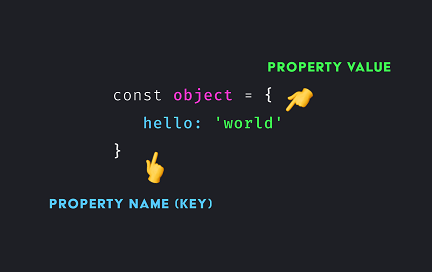# How to Get Property Values of JS Objects

JavaScript objects are key-value pairs that allows you to easily store different types of data in one place. Its data format is similar to JSON and is often used by web developers to transfer data to and from back end web servers. Sometimes you may need to get property values of JS objects. In this article, we will learn how to do this. There are several ways to do this. We will learn some of the simple and easy ones.

## How to Get Property Values of JS Objects

Let us say you have the following JS object.

`test = {'a':1, 'b':2}`

## 1. Using For..In Loop

One of the simplest ways to get property values of JS objects is using for…in loop. We just loop through the object and retrieve its value using the key, in each iteration.

```for(var key in test) {
var value = test[key];
}```

## 2. Using Object.values

Since 2017, most web browsers support Object.values property that directly returns an array of values.

```values = Object.values(test); //array of values

OR

for (val of Object.values(test)) {
// use individual value
}```

If you want both key and value from Object, use Object.entries() property.

```for (const [key, val] of Object.entries(test)) {
// use key and val
}```

## 3. For Older browsers

If you are using a web browser older than 2015, you can get list of keys using Object.keys() function and then use them to retrieve Object keys.

```var keys = Object.keys(test);

for (var i = 0; i < keys.length; i++) {
var val = test[keys[i]];
// use val
}

Object.keys(test).forEach(function (key) {
var val = test[key];
// use val
});```

If you want an array of object values, you can use the following code instead, which returns an array vals.

```var vals = Object.keys(test).map(function (key) {
return test[key];
});```

In this article, we have learnt how to get property values of JS object. You can use any of them as per your requirement.